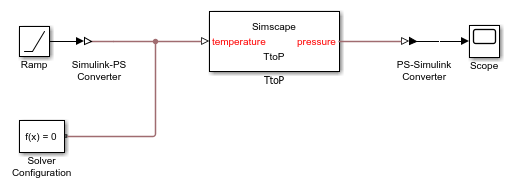## Using Lookup Tables in Equations

You can use the `tablelookup` function in the `equations` section of the Simscape™ file to interpolate input values based on a set of data points in a one-dimensional, two-dimensional, three-dimensional, or four-dimensional table. This functionality is similar to that of the Simulink® and Simscape Lookup Table blocks. It allows you to incorporate table-driven modeling directly in your custom block, without the need of connecting an external Lookup Table block to your model.

The following example implements mapping temperature to pressure using a one-dimensional lookup table.

```component TtoP inputs u = {0,'K'}; % temperature end outputs y = {0,'Pa'}; % pressure end parameters xd = {[100 200 300 400] 'K'}; yd = {[1e5 2e5 3e5 4e5] 'Pa'}; end equations y == tablelookup(xd,yd,u,interpolation=linear,extrapolation=nearest); end end```

`xd` and `yd` are declared as parameters with units. This enables the block users to provide their own data sets when the component is converted to a custom block, and also to select commensurate units from the drop-downs in the custom block dialog box. The next illustration shows the dialog box of the custom block generated from this component.Note

To avoid repeating the same parameter declarations in each component that needs to use them in its `tablelookup` function, you can declare table data sets as domain parameters and propagate them to components for interpolation purposes. For more information, see Propagation of Domain Parameters.

The following rules apply to the one-dimensional arrays `xd` and `yd`:

• The two arrays must be of the same size.

• For smooth interpolation, each array must contain at least three values. For linear interpolation, two values are sufficient.

• The `xd` values must be strictly monotonic, either increasing or decreasing.

The TtoP component uses linear interpolation for values within the table range, but outputs the nearest value of `yd` for out-of-range input values. The following illustration shows a block diagram, where the custom TtoP block is used with a linear input signal changing from 0 to 1000, and the resulting output.See the `tablelookup` reference page for syntax specifics and more examples.